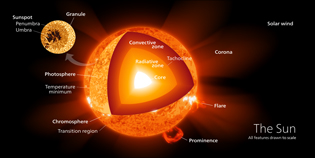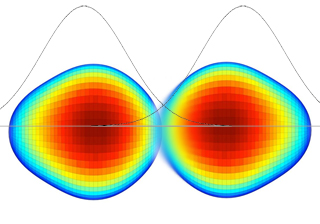# Where does the Sun's energy come from?

## Energy is at the center of the SunAutomatic translation Category: light and photons
Updated July 19, 2021

The Sun is a huge star with a diameter equal to 109 times that of the Earth, or 3.6 times the distance Earth/Moon. Its volume is equal to 1.3 million times that of the Earth.
The force gravitational at the surface (274 m/s2, 28 times that of the Earth) of this gigantic mass (1.989×1030 kg) contracts the matter in its center. Although his heart has a diameter equal to the Earth / Moon distance, the volume of the nucleus represents only 1.5% of the total volume.
Yet it is there, in his heart at about 15 million kelvins or degrees Celsius (1  k = -273.15 °C) that every second, 627 million tons of hydrogen merge to produce 622.7 million tons of helium. The difference in mass (4.3 million tons) is converted into energy, 4×1026 joules or about 1.5×1019 Kilowatt-hours (10 billion billion kw/h).
For 4.57 billion years, the Sun has consumed 4 million tons of hydrogen per second.

The gravitational pressure at the center of the Sun is 26 petapascals (26×1015 Pa), or 260 billion times the atmospheric pressure at the Earth's surface. At this pressure 1 m3 of hydrogen weighs 150 tons. 1 m3 of hydrogen on Earth weighs 90 grams. So there are 1.6 million times more hydrogen atoms in 1 m3 at the center of the Sun than in 1 m3 of hydrogen on earth (it's not that huge).
At this pressure (260 billion times atmospheric pressure) and at this temperature (15 million Kelvin) nuclear fusion can start.
The hydrogen nuclei will merge and form a helium nucleus, releasing a lot of energy (15 times more than the nuclear fission triggered in our power plants).## How to merge two protons?

For 2 protons to merge, the distance between them must be very small, the size of the nucleus (10-15 meter). For this, it is necessary to cross the Coulomb barrier (force of the electrical interaction between two positively charged protons). This force is proportional to the product of the two charges and inversely proportional to the square of the distance between the two charges (which requires considerable energy to « stick » two protons). The closer the protons get, the more energy is needed to « stick » them together. Even at 15 million Kelvin, the energy is not enough to cross the Coulomb barrier. Strong proton shocks do not crush strong nuclear interaction bubbles enough to « stick » 2 protons together.
Theoretically, protons would have to travel at 20,000 km/s to reach a temperature of 5 billion Kelvin. At 15 million Kelvin the protons move at 600 km/s.
However, the temperature of 15 million Kelvin is an average temperature. According to the distribution curve of the particles as a function of the Maxwell-Boltzmann temperature, the protons are far from reaching 20,000 km/s and therefore fusion is impossible.
To achieve fusion it is necessary to invoke quantum physics.
The size or position of a proton is a density of probability represented by a curve. The further away from the top of the curve, the less likely the presence of the proton or the size of the proton. At the bottom of the curve the probability of having 2 protons that merge is very small but not zero.
The property, which a quantum object has of crossing a potential barrier even if its energy is less than the minimum energy required for crossing this barrier is called the tunnel effect.

Crossing the Coulomb barrier is impossible at low temperature, but with an average of 15 million Kelvin, this is enough for the tunnel effect to become probable without having to reach 5 billion Kelvin.
Every second a proton suffers 2x1015 collisions and the tunnel effect occurs once every 108 collisions. So each proton has 20 million opportunities to merge.
So why don't all of the Sun's protons fuse together in a second?
Because 2 protons although united by the strong nuclear interaction, cannot coexist because of the exclusion principle of Wolfgang Ernst Pauli (1900 - 1958). Thus each proton merges with another proton 20 million times per second thanks to the tunnel effect and separates instantly (10-21 second).
To make a helium nucleus it merges 2 protons and 2 neutrons.
Where do neutrons come from?
Weak nuclear interaction (radioactivity) will intervene in our fusion. Nuclear interaction weak (100,000 times weaker than the strong nuclear interaction) allows a proton, with an extremely low probability (1 in 1024) to transform into a neutron when it is in contact with another proton. When this happens a deuterium nucleus appears emitting a neutrino and a positron which immediately annihilates with a surrounding electron transforming matter in pure energy (E=mc2).
The deuterium will transform into helium 3 emitting according to the same principle of pure energy (gamma ray). Always with a low probability then 2 cores helium 3 will give 2 helium 4 nuclei.
This process called the « proton-proton chain » thanks to a very low probability of fusion has been maintained for 4.6 billion years.### Image: The size or position of a proton is a probability density represented by a curve. The further away from the top of the curve, the less the presence of the proton or the size of the proton is likely. However, at the bottom of the curve, the probability of having 2 protons merging is low but not zero. For the nuclear reaction « to ignite », it is necessary that protons (hydrogen nuclei) come into contact. Then one of the two protons turns into a neutron. Without radioactivity, this transformation would be impossible. Two protons, even in contact, are unable to cross the Coulomb barrier, they push back. But the proton can transiently transform into a neutron by emitting a particle (W boson). This W boson is immediately reabsorbed, the neutron becoming proton again. Very rarely, it happens that the W boson decays into a positron and a neutrino and at this point, the neutron remains a neutron. It can then merge with the other proton to form a nucleus of deuterium and switch on the « proton-proton chain ».

 1997 © Astronoo.com − Astronomy, Astrophysics, Evolution and Earth science. Contact    Mentions légales